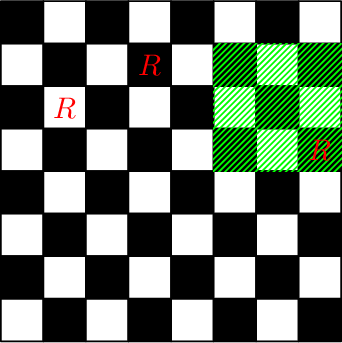2022-05-15 21:11:31

## 题意

• 在第 $x$ 行第 $y$ 列的位置，放置一个 Rook 棋子
• 移除在第 $x$ 行第 $y$ 列的位置已有的 Rook 棋子
• 判断在 $(x1, y1)$ 到 $(x2, y2)$ 范围内所有的位置，是否全部可以被 Rook 棋子攻击？

$n \leq 2 \times 10^5$。

## 分析struct Fenwick {
int n;
std::vector<int> v;
Fenwick(int n) : n(n), v(n + 1) {}
int lowbit(int x) { return x & -x; }
void add(int x, int y) {
for (; x <= n; x += lowbit(x)) v[x] += y;
}
int sum(int x) {
int res = 0;
for (; x; x -= lowbit(x)) res += v[x];
return res;
}
int rangeSum(int l, int r) { return sum(r) - sum(l - 1); }
};

void solution() {
int n, q;
std::vector<int> row(n + 1, 0), col(n + 1, 0);
Fenwick bitRow(n), bitCol(n);
while (q--) {
int opt;
if (opt == 1) {
int x, y;
// a[x][y] = true;
++row[x];
++col[y];
if (row[x] == 1) bitRow.add(x, 1);
if (col[y] == 1) bitCol.add(y, 1);
} else if (opt == 2) {
int x, y;
// a[x][y] = false;
--row[x];
--col[y];
if (row[x] == 0) bitRow.add(x, -1);
if (col[y] == 0) bitCol.add(y, -1);
} else {
int x1, y1, x2, y2;
}